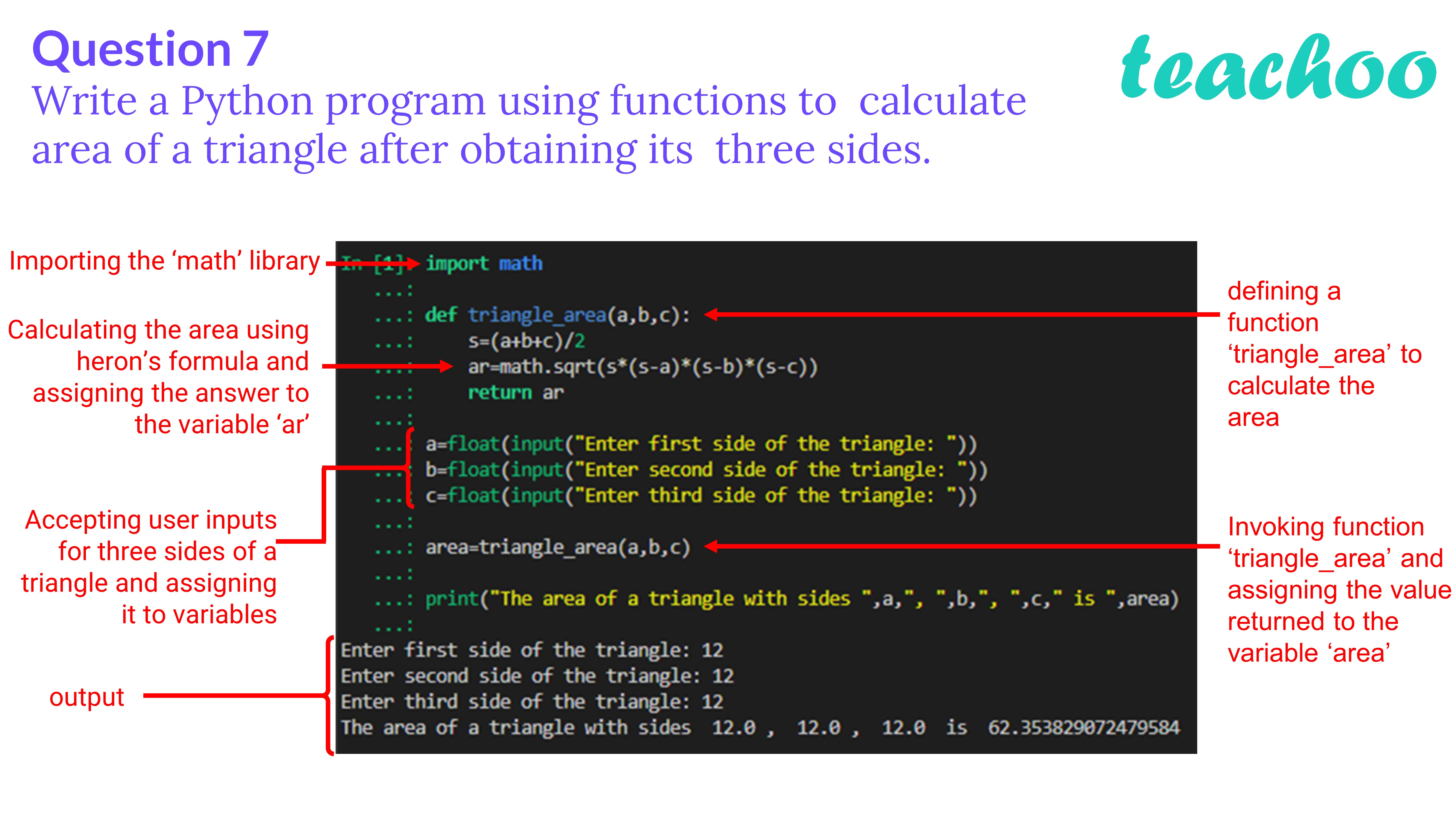Past Year - 2 Mark Questions

Computer Science - Class 12
Chapter 14 - Functions in python

## Write a Python program using functions to calculate area of a triangle after obtaining its three sides.

``` import math ```

``` def triangle_area (a,b,c): ```

```     s=(a+b+c)/ 2 ```

```     ar=math.sqrt(s*(s-a)*(s-b)*(s-c)) ```

```     return ar ```

``` a=float(input( "Enter first side of the triangle: " )) ```

``` b=float(input( "Enter second side of the triangle: " )) ```

``` c=float(input( "Enter third side of the triangle: " )) ```

``` area=triangle_area(a,b,c) ```

``` print("The area of a triangle with sides ",a,", ",b,", ",c," is ",area) ```Learn in your speed, with individual attention - Teachoo Maths 1-on-1 Class

### Transcript

Question 7 Write a Python program using functions to calculate area of a triangle after obtaining its three sides. Importing the ‘math’ library Calculating the area using heron’s formula and assigning the answer to the variable ‘ar’ Accepting user inputs for three sides of a triangle and assigning it to variables output defining a function ‘triangle_area’ to calculate the area Invoking function ‘triangle_area’ and assigning the value returned to the variable ‘area’